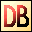DelphiBasics
 TanFunction The Tangent of a number Math unit
 function Tan(const Number Extended):Extended;
Description
The Tan function is a mathematical function giving the Tangent value of a Number value given radians.

Notes
The System unit supports only the following angular functions:

ArcTan
Sin
Cos

Related commands
 ArcCos The Arc Cosine of a number, returned in radians ArcSin The Arc Sine of a number, returned in radians ArcTan The Arc Tangent of a number, returned in radians Cos The Cosine of a number Sin The Sine of a numberDownload this web site as a Windows program.

 Example code : Get the Tangent of 45 degrees var   float : single; begin   // The Tangent of 45 degrees = 1.0   float := Tan(PI/4);  // = 180/4 = 45 degrees   ShowMessage('Tan(PI/4) = '+FloatToStr(float)); end; Show full unit code Tan(PI/4) = 1